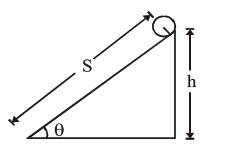Deepak Scored 45->99%ile with Bounce Back Crack Course. You can do it too!

# The following bodies,

Question:

The following bodies,

(1) a ring

(2) a disc

(3) a solid cylinder

(4) a solid sphere,

of same mass ' $m$ ' and radius ' $R$ ' are allowed to roll down without slipping simultaneously from the top of the inclined plane. The body which will reach first at the bottom of the inclined plane is

[Mark the body as per their respective numbering given in the question]Solution:

$M g \sin \theta R=\left(m k^{2}+m R^{2}\right) \alpha$

$\alpha=\frac{\mathrm{Rg} \sin \theta}{\mathrm{k}^{2}+\mathrm{R}^{2}} \Rightarrow a=\frac{\mathrm{g} \sin \theta}{1+\frac{\mathrm{k}^{2}}{\mathrm{R}^{2}}}$

$t=\sqrt{\frac{2 s}{a}}=\sqrt{\frac{2 s}{g \sin \theta}\left(1+\frac{k^{2}}{R^{2}}\right)}$

for least time, $\mathrm{k}$ should be least \& we know $\mathrm{k}$ is least for solid sphere.